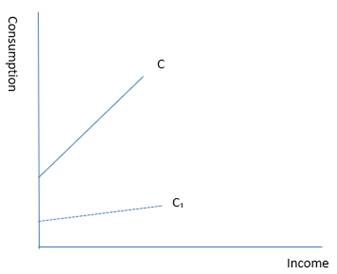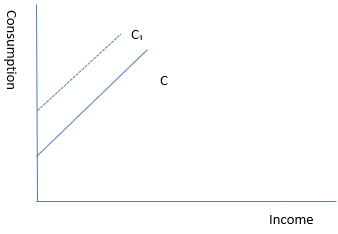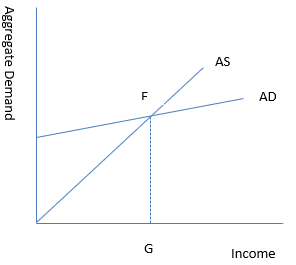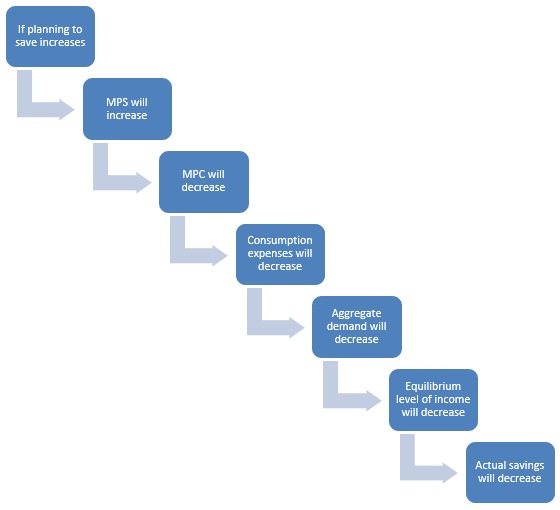### Income Determination Class 12th Introductory Macroeconomics CBSE Solutio

##### Question 1.What is marginal propensity to consume? How is it related to marginal propensity to save?Answer:Marginal propensity to consume – It is the proportion of additional income going to consumption expenditure. It is the ratio between change in consumption and change in income.Marginal propensity to save – It is the proportion of additional income that goes to saving. It is the ratio between change in savings and change in incomePropensity to consume means desire to consume so marginal propensity to consume is the ratio of increase in consumption due to increase in income. In the same way propensity to save means desire to save, so marginal propensity to save means the ratio of increase in saving due to increase in income.Relation between Marginal Propensity to Consume (MPC) and Marginal Propensity to Save (MPS)MPC = ∆C/∆YMPS = ∆S/∆YWhere∆C = Change in Consumption∆Y = Change in Income∆S = Change in SavingMPC + MPS = 1Y = C + S∆Y = ∆C + ∆SDividing both sides by ∆Y∆Y/∆Y = ∆C/∆Y + ∆S/∆Y1 = MPC + MPSØ MPC = 1 – MPS, ORØ MPS = 1 – MPCExample - Suppose the income increases from 200 crores to 250 crores and consumtion increses from 20 crores to 40 crores.∆Y = 50 crores∆C = 20 croresMPC = 20/50 = 40%MPS = 1 – 40% = 60%This means that if 40% of change in income is going into consumption then the remaining 60% will go to saving.Question 2.What is the difference between ex ante investment and ex post investment?Answer:Ex ante investment is the planned investment and ex post investment is the actual investment.Planned investment is investment which is desired to be made by firms and planners in economy during the year whereas actual or realised investment is the investment made during that period.According to Keynes investment in inventory of unsold goods is unplanned investment so actual investment is the sum of planned and unplanned investment.Unplanned investment is that investment which was not included in planned investment.Question 3.What do you understand by ‘parametric shift of a line’? How does a line shift when its(i) slope decreases, and (ii) its intercept increases?Answer:Change in consumption curve due to the change in its slope is known as parametric shift in the curve.Let C = C + by be the consumption functionWhere,b – MPCy – Income(i) When slope decreases – The consumption curve will swing downwards. This is known as parametric shift in the curve.(ii) When its intercept increases – Intercept is the autonomous part of consumption function, so when intercept increases there will be a parallel shift in the consumption curve.Question 4.What is ‘effective demand’? How will you derive the autonomous expenditure multiplier when price of final goods and the rate of interest are given?Answer:When aggregate demand is at the point of equilibrium it is called effective demand because at this point it becomes effective in determining the national income. Effective demand is that level of demand which is fully made by corresponding supply and there is no tendency of expansion and contraction.According to Keynesian theory, in short run the equilibrium level of income and employment is determined by the level of effective demand because aggregate supply or national income is assumed to be given and constant at that level.E is the equilibrium point where the aggregate supply (AS) curve and aggregate demand (AD) curve meet, EG is the effective demand and output level is determined by ADAutonomous expenditure multiplierY = AD (at equilibrium)AD = A + cYY = A + cYY - cY = AY (1 - c) = AY = A / (1 – c)Where,A = Autonomous expenditurec = MPCY = level of incomeA / (1 – c) = autonomous expenditure multiplierSo, the autonomous expenditure multiplier is dependent on the income and MPC.Question 5.Measure the level of ex-ante aggregate demand when autonomous investment and consumption expenditure (A) is Rs 50 crores, and MPS is 0.2 and level of income (Y) is Rs 4000 crores. State whether the economy is in equilibrium or not (cite reasons).Answer:MPC = 1 – MPS= 1 – 0.2= 0.8Y = 4000 croresAD = C + I = C0 + CY + I = C0 + I + CYAD = A + CYWhere,A = C0 + I= 50 + 0.8 (4000)= 3250crAS (Y) = 4000crHere aggregate demand is less than aggregate supply so the economy is not in equilibrium this means that the buyers are planning to buy less than what the sellers are planning to produce and sell, due to this the inventories will accumulate. So the producers will reduce the production level and workers will be laid off.Question 6.Explain ‘Paradox of Thrift’.Answer:The growth of a country is dependent upon its level of investment and level of investment depends upon the level of savings that is thrift or savings lead to increased income in the economy.According to Prof. Keynes, the increase in savings in the economy may not benefit the economy as a whole because if all people will increase the proportion of income they save, the total value of savings will not increase. It will either decline or remain unchanged.The paradox of thrift states that as people become thriftier, they end up saving less or same as before.With help of following chart we can understand it easilyPDF FILE TO YOUR EMAIL IMMEDIATELY PURCHASE NOTES & PAPER SOLUTION. @ Rs. 50/- each (GST extra)

HINDI ENTIRE PAPER SOLUTION

MARATHI PAPER SOLUTION

SSC MATHS I PAPER SOLUTION

SSC MATHS II PAPER SOLUTION

SSC SCIENCE I PAPER SOLUTION

SSC SCIENCE II PAPER SOLUTION

SSC ENGLISH PAPER SOLUTION

SSC & HSC ENGLISH WRITING SKILL

HSC ACCOUNTS NOTES

HSC OCM NOTES

HSC ECONOMICS NOTES

HSC SECRETARIAL PRACTICE NOTES

# 2019 Board Paper Solution

HSC ENGLISH SET A 2019 21st February, 2019

HSC ENGLISH SET B 2019 21st February, 2019

HSC ENGLISH SET C 2019 21st February, 2019

HSC ENGLISH SET D 2019 21st February, 2019

SECRETARIAL PRACTICE (S.P) 2019 25th February, 2019

HSC XII PHYSICS 2019 25th February, 2019

CHEMISTRY XII HSC SOLUTION 27th, February, 2019

OCM PAPER SOLUTION 2019 27th, February, 2019

HSC MATHS PAPER SOLUTION COMMERCE, 2nd March, 2019

HSC MATHS PAPER SOLUTION SCIENCE 2nd, March, 2019

SSC ENGLISH STD 10 5TH MARCH, 2019.

HSC XII ACCOUNTS 2019 6th March, 2019

HSC XII BIOLOGY 2019 6TH March, 2019

HSC XII ECONOMICS 9Th March 2019

SSC Maths I March 2019 Solution 10th Standard11th, March, 2019

SSC MATHS II MARCH 2019 SOLUTION 10TH STD.13th March, 2019

SSC SCIENCE I MARCH 2019 SOLUTION 10TH STD. 15th March, 2019.

SSC SCIENCE II MARCH 2019 SOLUTION 10TH STD. 18th March, 2019.

SSC SOCIAL SCIENCE I MARCH 2019 SOLUTION20th March, 2019

SSC SOCIAL SCIENCE II MARCH 2019 SOLUTION, 22nd March, 2019

XII CBSE - BOARD - MARCH - 2019 ENGLISH - QP + SOLUTIONS, 2nd March, 2019

# HSCMaharashtraBoardPapers2020

(Std 12th English Medium)

HSC ECONOMICS MARCH 2020

HSC OCM MARCH 2020

HSC ACCOUNTS MARCH 2020

HSC S.P. MARCH 2020

HSC ENGLISH MARCH 2020

HSC HINDI MARCH 2020

HSC MARATHI MARCH 2020

HSC MATHS MARCH 2020

# SSCMaharashtraBoardPapers2020

(Std 10th English Medium)

English MARCH 2020

HindI MARCH 2020

Hindi (Composite) MARCH 2020

Marathi MARCH 2020

Mathematics (Paper 1) MARCH 2020

Mathematics (Paper 2) MARCH 2020

Sanskrit MARCH 2020

Sanskrit (Composite) MARCH 2020

Science (Paper 1) MARCH 2020

Science (Paper 2)

Geography Model Set 1 2020-2021

MUST REMEMBER THINGS on the day of Exam

Are you prepared? for English Grammar in Board Exam.

Paper Presentation In Board Exam

How to Score Good Marks in SSC Board Exams

Tips To Score More Than 90% Marks In 12th Board Exam

How to write English exams?

How to prepare for board exam when less time is left

How to memorise what you learn for board exam

No. 1 Simple Hack, you can try out, in preparing for Board Exam

How to Study for CBSE Class 10 Board Exams Subject Wise Tips?

JEE Main 2020 Registration Process – Exam Pattern & Important Dates

NEET UG 2020 Registration Process Exam Pattern & Important Dates

How can One Prepare for two Competitive Exams at the same time?

8 Proven Tips to Handle Anxiety before Exams!